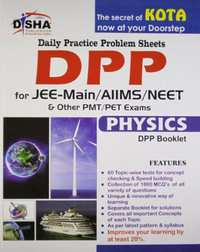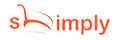Share

# Daily Practice Problem (DPP) Sheets for JEE Main/AIIMS/NEET Physics (Kota's Formula to Success) NEET (UG)M.R.P 350/-220.00(37%)
Product Description

Daily Practice Problem (DPP) Sheets for JEE Main or AIIMS or NEET Physics (Kota's formula to Success)

Assessment is the most integral part of a student's preparation but still most of them avoid it. Assessment can only tell where you stand and how you can improve from that point. So it is very important that you take the right assessment, which is on the correct pattern, has the same level of difficulty as the actual exam and covers all the important concepts of the subject. Disha Publication launches a first of its kind product which changed the way coaching was conducted in Kota - the hub of Engineering and Medical Entrance education in India.

The book "Daily Practice Problem (DPP) Sheets for JEE Main or NEET Physics" is precise, apt and tuned to all the requirements of a JEE Main aspirant. No matter where you prepare from - a coaching or NCERT books or any other textbook or Guide - Daily Practice Problem Sheets provides you the right assessment on each topic. Your performance provides you the right cues to improve your concepts so as to perform better in the final examination.

It is to be noted here that these are not tests but act as a checklist of student's learning and ability to apply concepts to different problems. It is our strong belief that if an aspirant works hard on the cues provided through each of the DPP sheets he or she can improve his or her learning and finally the score by at least 20%.

• DPP 1. Physical World, Units and Dimensions
• DPP 2. Measurements (Errors)
• DPP 3. Motion in A Straight Line 1 (Distance, Displacement, Uniform and Non-uniform motion)
• DPP 4. Motion in A Straight Line 2 (Relative Motion and Motion Under Gravity)
• DPP 5. Vectors
• DPP 6. Motion in A Plane-1 (Projectile Motion)
• DPP 7. Motion in Plane-2 (Horizontal Circular Motion)
• DPP 8. Motion in Plane-3 (Vertical Circular Motion, Relative Motion)
• DPP 9. Laws of Motion-1 (Newton's third laws, momentum, pseudo force concept)
• DPP 10. Laws of Motion-2 (Blocks in contact, connected by string, pulley arrangement)
• DPP 11. Laws of Motion-3 (Friction)
• DPP 12. Work, Energy and Power-1 (Work by constant and variable forces, kinetic and potential energy, work energy theorem)
• DPP 13. Work, Energy and Power-2 (Conservation of momentum and energy, collision, rocket case)
• DPP 14. Centre of mass and its motion
• DPP 15. Rotational Motion 1: Basic concepts of rotational motion, moment of a force, torque, angular momentum and its conservation with application
• DPP 16. Rotational Motion-2 : Moment of inertia, radius of gyration, (values of moments of inertia simple geometrical objects)
• DPP 17. Rolling Motion (Parallel and perpendicular theorems and their applications, Rigid body rotation, equations of rotational motion)
• DPP 18. Gravitation - 1 (The Universal law of gravitation, Acceleration due to gravity and its variation with altitude and depth, Kepler's law of planetary motion)
• DPP 19. Gravitation - 2 (Gravitational potential energy, Gravitational potential, Escape velocity and Orbital velocity of a satellite, Geo-stationary satellites)
• DPP 20. Elasticity
• DPP 21. Fluid Mechanics
• DPP 22. Thermal Expansion-1 (Thermal Expansion, Calorimetry and Change of State)
• DPP 23. Thermal Expansion-2 (Heat transfer and Newton's law of cooling)
• DPP 24. Thermodynamics-1 (Thermal equilibrium, zeroth law of thermodynamics, concept of temperature, Heat, work and internal energy, Different processes)
• DPP 25. Thermodynamics-2 (1st and 2nd laws of thermodynamics reversible and irreversible processes, Carnot engine and its efficiency)
• DPP 26. Kinetic Theory
• DPP 27. Oscillations-1 (Periodic motion - period, Frequency, Displacement as a function of time. Periodic functions, Simple harmonic motion and its equation, Energy in S.H.M. - kinetic and potential energies)
• DPP 28. Oscillations-2 (Oscillations of a spring, simple pendulum, free, forced and damped oscillations, Resonance)
• DPP 29. Waves-1 (Wave motion, longitudinal and transverse waves, speed of a wave, displacement relation for a progressive wave, principle of superposition of waves, reflection of waves)
• DPP 30. Waves-2 (Standing waves in strings and organ pipes, Fundamental mode and harmonics, Beats, Doppler effect in sound)
• DPP 31. Practical Physics - 1
• DPP 32. Electrostatics-1 (Coulomb's law, electric field, field lines, Gauss's law)
• DPP 33. Electrostatics-2 (Electric potential and potential difference, equipotential surfaces, electric dipole)
• DPP 34. Electrostatics -DPP 3 (Electrostatic Potential energy, conductors)
• DPP 35. Electrostatics-4 (Capacitors, dielectrics)
• DPP 36. Current Electricity - 1 (Electric Current, drift velocity, Ohm's law, Electrical resistance, Resistances of different materials, V-I characteristics of Ohm and non-ohmic conductors, electrical energy and power, Electrical resistivity, Colour code of resistors, Temperature dependence of resistance
• DPP 37. Current Electricity-2 : Electrical cell and its internal resistance, Potential difference and E.M.F of a cell, Combination of cells in series and in parallel, Kirchoff's laws and their applications, RC transient circuit, Galvanometer, Ammeter, Voltmeter
• DPP 38. Current Electricity-
• DPP 3 : Wheatstone bridge, Meter bridge, Potentiometer-principle and its applications.
• DPP 39. Magnetic Effects of Current-1 (Magnetic field due to current carrying wires, Biot savart law)
• DPP 40. Magnetic Effects of Current-2 : (Motion of charge particle in a magnetic field, force between current carrying wires.)
• DPP 41. Magnetic Effects of Current-DPP 3 (Magnetic dipole, Current carrying loop in magnetic field, Galvanometer )
• DPP 42. Magnetism and Matter - 1 (Bar magnet as an equivalent solenoid, Magnetic field lines, Earth's magnetic field and magnetic elements)
• DPP 43. Magnetism and Matter-2 (Para, dia and ferro-magnetic substances, magnetic susceptibility and permeability, Hysteresis, Electromagnets and permanent magnets.)
• DPP 44. Electromagnetic induction-1 (Magnetic flux, Faraday's law of electromagnetic induction, Lenz's law, motional e.m.f.)
• DPP 45. Electromagnetic induction - 2 : Self inductance, mutual inductance, Growth and decay of current in L.R. circuit, Transformer, Electric motor, Generator
• DPP 46. Alternating Current - 1 (Alternating currents, peak and rms value of alternating current or voltage, reactance and impedance, Pure circuits, LR, CR ac circuits.)
• DPP 47. Alternating Current - 2 (LCR series circuit, resonance, quality factor, power in AC circuits, wattles and power current)
• DPP 48. EM Waves
• DPP 49. Ray Optics-1 (Reflection on plane mirrors and curved mirrors)
• DPP 50. Ray Optics - II (Refraction on plane surface, total internal reflection, prism)
• DPP 51. Ray Optics - DPP 3 (Refraction on curved surface lens, Optical instrument)
• DPP 52. Wave Optics - I (Interference of Light)
• DPP 53. Wave Optics - II : Diffraction and polarisation of light
• DPP 54. Dual Nature of Matter and Radiation (Matter Waves, Photon, Photoelectric effect, X-ray)
• DPP 55. Atoms
• DPP 56. Nuclei
• DPP 57. Semiconductor Electronics - 1 (Semiconductors, LED, Photodiode, Zener diode)
• DPP 58. Semiconductor Electronics-2 (Junction transistor, transistor action, characteristics of a transistor, transistor as an amplifier, logic gates)
• DPP 59. Communication Systems, Laser
• DPP 60. Practical Physics - 2